• 设计一个双向冒泡排序算法。要求用C/C++实现。数据结构
• 主要介绍了Java实现冒泡排序与双向冒泡排序算法的代码示例,值得一提的是所谓的双向冒泡排序并不比普通的冒泡排序效率来得高,注意相应的时间复杂度,需要的朋友可以参考下
• 本文实例为大家分享了C++实现双向冒泡排序算法的具体代码，供大家参考，具体内容如下 一、概念（来源于百度百科） 传统冒泡算法原理 冒泡排序算法的运作如下：（从后往前） 1.比较相邻的元素。如果第一个比第二个大...
• 主要介绍了浅析java双向冒泡排序算法,并附上源码，需要的朋友可以参考下
• 冒泡排序算法应该算是每个开发者入门必学的基础算法，它逻辑清晰简单，代码实现也并不复杂，这里用自己的话语来总结一下。 （这些为了方便随便打开了一个node项目写的，其他Java ，PHP，C等也是一样的道理，算法讲究...
冒泡排序算法应该算是每个开发者入门必学的基础算法，它逻辑清晰简单，代码实现也并不复杂，这里用自己的话语来总结一下。
（这些为了方便随便打开了一个node项目写的，其他Java ，PHP，C等也是一样的道理，算法讲究的只是一个思维模式，知道怎么去实现就行，不要死抠语言）
先说简单的普通冒泡排序：
冒泡排序既然是一个排序算法，那么它就一定是有一个循环排序的过程，其实它的原理很简单，就是两层for循环嵌套，外层for循环控制总的循环次数，内层则是不断拿前一个值和后一个值去比较，不断把小的放前面（当然你也可以把大的放前面）。那么这里有几个坑点是要注意的：
坑点1:外层for循环要记得下标和数组长度的对应，下标从0开始，那么0～9就有十个数了，千万别用正常计数思维想着只有九个数，在用.length方法的时候要注意，不然在最后一轮循环会出现undefined拿不到值或者null point之类的错误。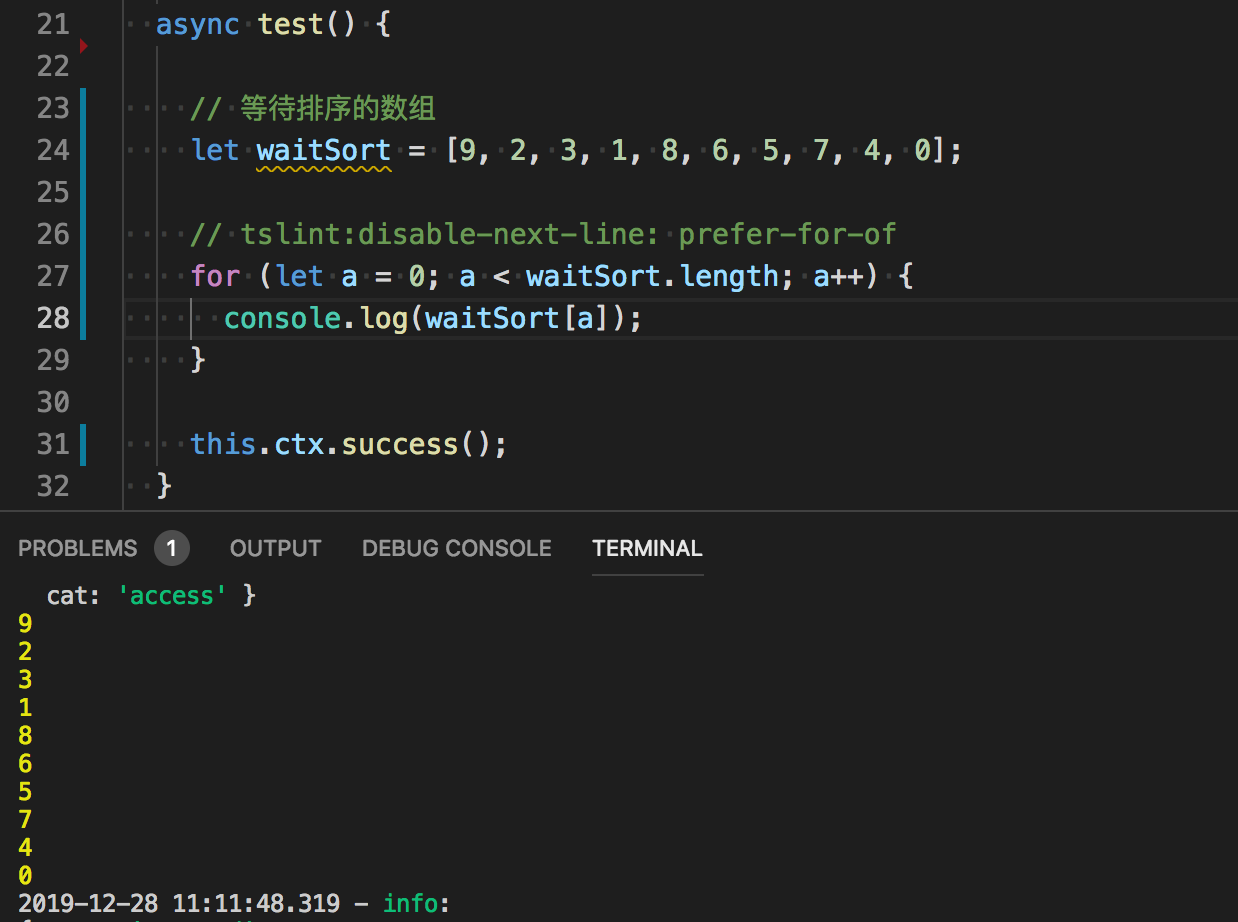比如这里数组长度0～9乱序，就是有十个数，所以 list.length 长度就是10，在写判断条件时候如果写a <=  list.length还跑循环的话，就会出现undefind了。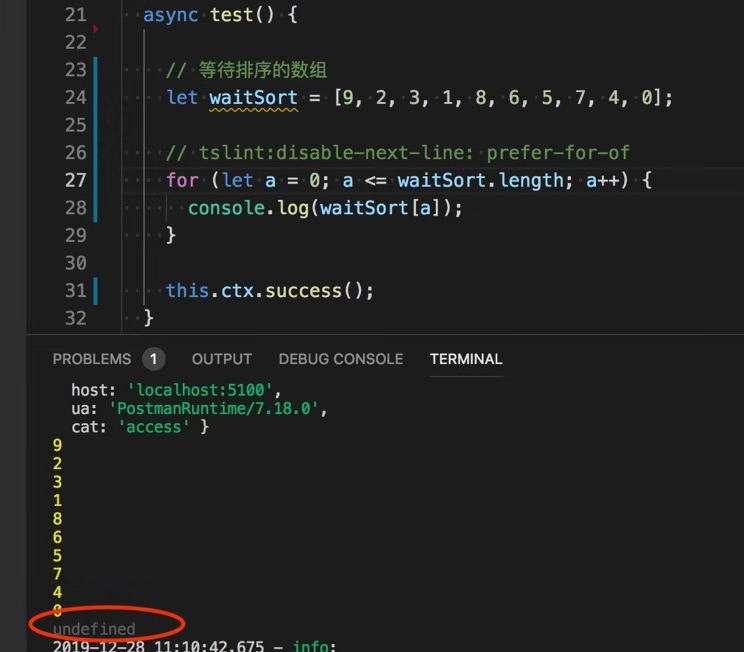坑点2:很多初学者在两个数交换位置那里有点疑惑，这个是很简单一个数据交换小问题，就是要定义一个容器去装。两杯水互换，需要第三个临时容器一样的道理，交换的时候记得是数组下标的值互换，不是从数组对应下标拿出的值互换（那样子没改变数组下标元素），起不到重新排序效果。
错误示范：let tempBox;
let a = a[x];
let b = b [x];
if (a > b ) {
tempBox = a;
a = b;
b = tempBox;
}
乍看之下没问题啊，互换啊，为啥排序完跟没排序一样？？那就是你只交换一个赋值后的变量，根本的数组对应下标的值你都没发生改动！！
正确示范：
if (waitSort[a] > waitSort[b]) {
tempBox = waitSort[a];
waitSort[a] = waitSort[b];
waitSort[b] = tempBox;
}
坑点三：记住外圈循环是从拿第一个元素开始，内圈循环是从第二个开始往后拿，当到第二轮循环时候，外圈从拿第二个元素开始，那么内圈就是第三个元素开始往后拿，也就是说，外圈是n开始，内圈循环是n+1开始一直往后拿！切记内圈的任务是拿剩下还没排序的进行排序而已。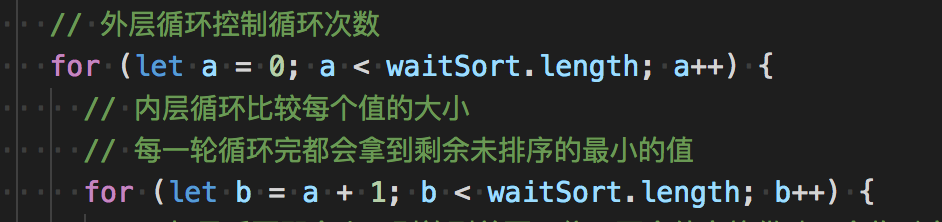坑点四：学算法光靠看和复制粘贴跑一次是学不懂的，一定要自己拿纸拿笔推演一遍！推演两遍三遍彻底明白它怎么实现的一个逻辑，自己再用代码去实现！这是最重要的一点坑！算法不能靠背诵！
下面上源码给大家：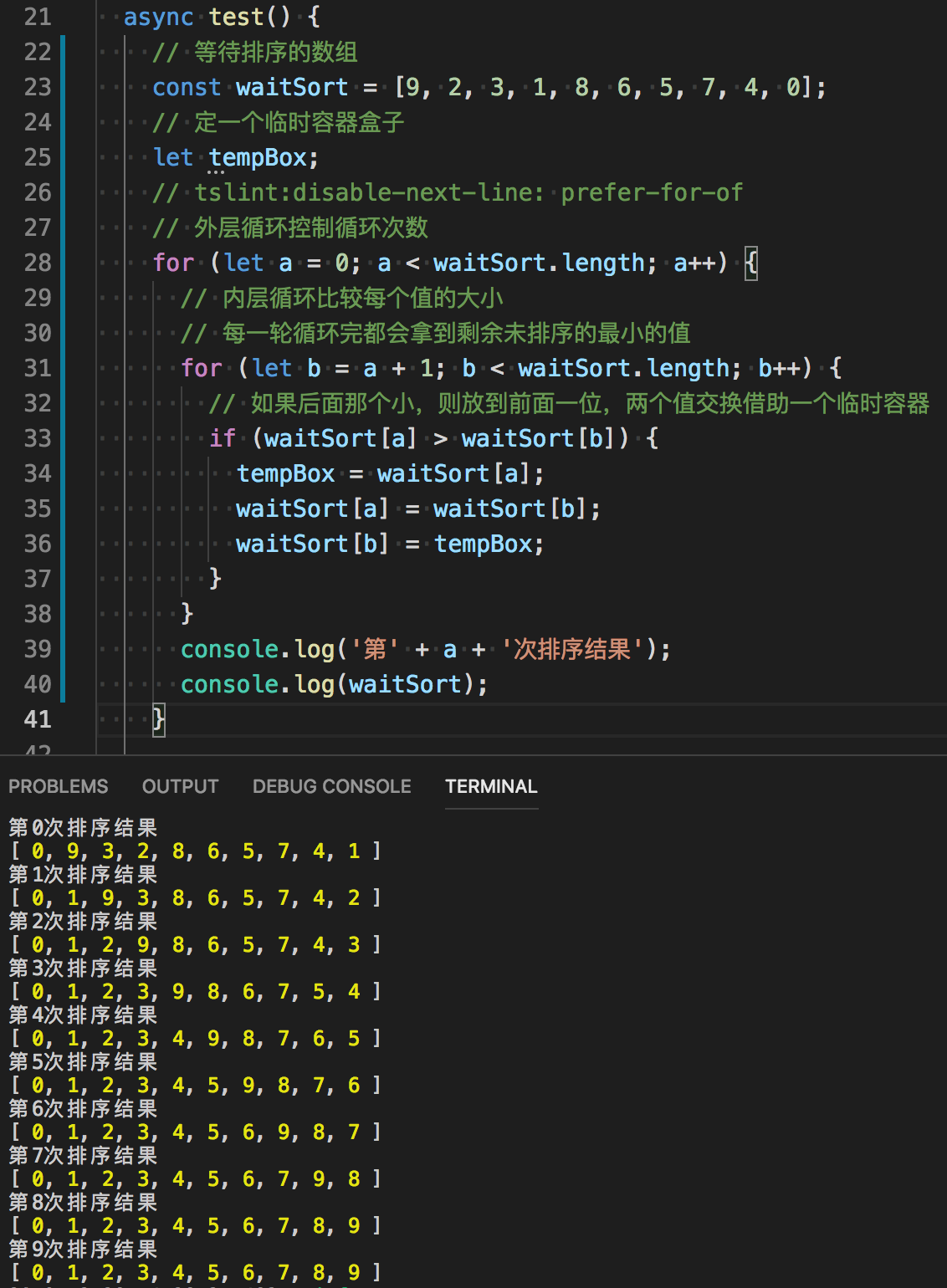流程解释：
1:外层先拿下标0的第一个元素9。内层开始比较9是不是最小的，内层一个个比较循环下来发现0才是最小的，于是放0在第一位，9在跟2比较的时候就被比下去了，所以被丢在2那个下标位置，2跟1比较时候又被丢下在1那个位置，拿着1去继续比较，1跟0PK的时候被丢下在0的位置，0上升到一开始9所在的下标位置。
2：外层再拿下标1的元素丢进内循环比较。然后继续上一步的循环比较，把大的丢在当前下标位置，小的拿着继续比较，内循环结束，拿到最小的值1丢在下标为1的位置。
3:接下来都是一样的操作，这里就不解释了。（如果看不懂，那就很正常，赶紧动手动笔画一画流程！！花不了十分钟！不然你看谁的都看不懂！！）
从控制台我们可以很清晰看到，每一次循环，都会把剩余的未排序的数组中最小的一个元素放到最前面，像汽水冒泡一样一个个冒泡上来，这就是简单的普通冒泡排序算法。
冒泡排序的时间复杂度（也就是花的时间）为：O(n²)，最好的情况下是O(n)；空间复杂度（也就是占用内存空间的大小）是O(1)，属于稳定的排序算法。
下面说双向冒泡排序：
双向冒泡排序要比普通冒泡难，但是它的速度会更快，在数据量大的情况下，速度差距是几倍的，因为双向冒泡排序减少了for循环，并且两边已经排好序的数组元素在下一次循环中不会参与到比较，大大减少了很多无用操作。网上关于双向冒泡的资源很多，这里我根据个人的理解来进行讲解和分享源码展示。
双向冒泡其实就是在一次循环中进行两次内圈循环，分别找出最大的元素放到最后和找出最小的元素放到最前面。同时进行从前往后排和从后往前排，最后到达两者交汇点就证明全部数组元素都已经正确进行了排序（反之如果头尾两者没有相等，还是头 小于 尾，则证明头尾之间还夹着没排序好的元素）；难点在于理清开始和结束的下标，因为记录前后下标每一次循环结束都会改变，下一次循环会直接从上一次排好序的下标位置开始对中间没有排序部分进行处理。（可能这样说很难理解，实际上真的是比较难理解，一定要自己动手画图实现，一定要自己理解后用代码再实现一遍！！）
首先是从前往后找部分，把最大的一个放到最后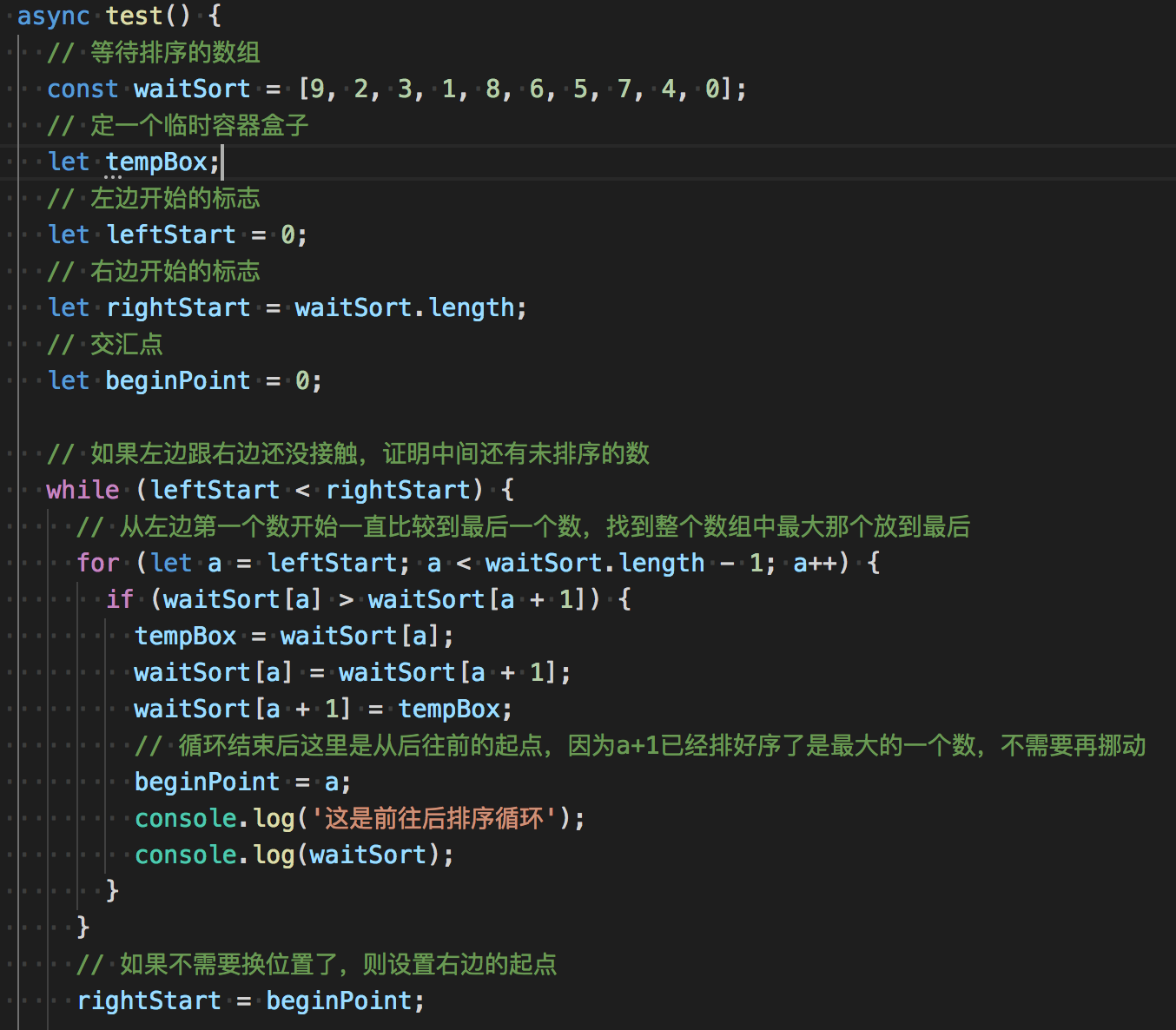接下来就是后往前找部分，找出未排序中最小的一个放到数组的前面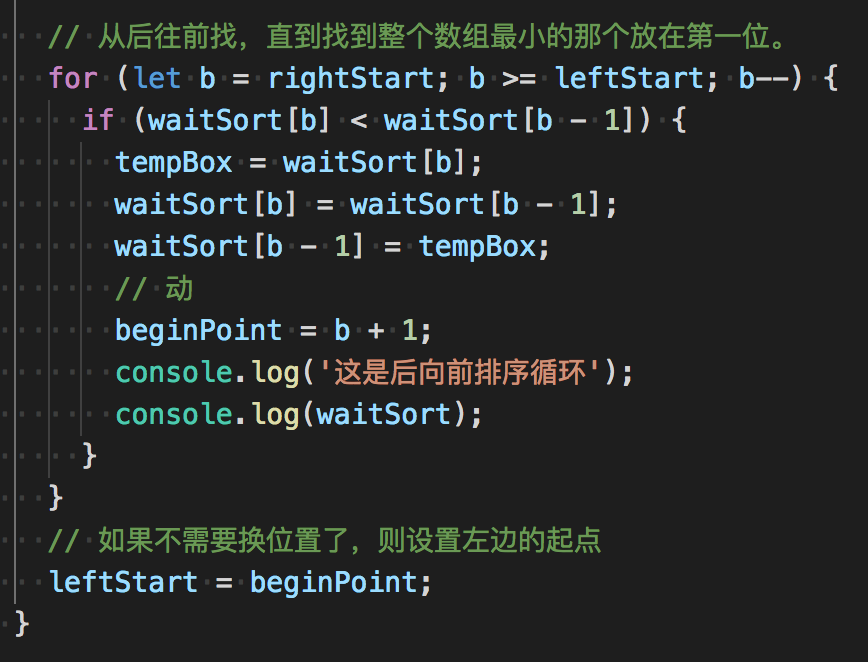执行结果：
请重点看第一轮的9和第二轮的0，它们都是乱序中的最大和最小，它们都能够自觉的跑到自己该呆的位置，然后从此退出江湖，不再参与比较大小，而夹在它们二者中间那些乱序的，还迷茫的元素们，还是会进行不断的循环比较排序。直到rigthStart = leftStart  头尾相连的时候。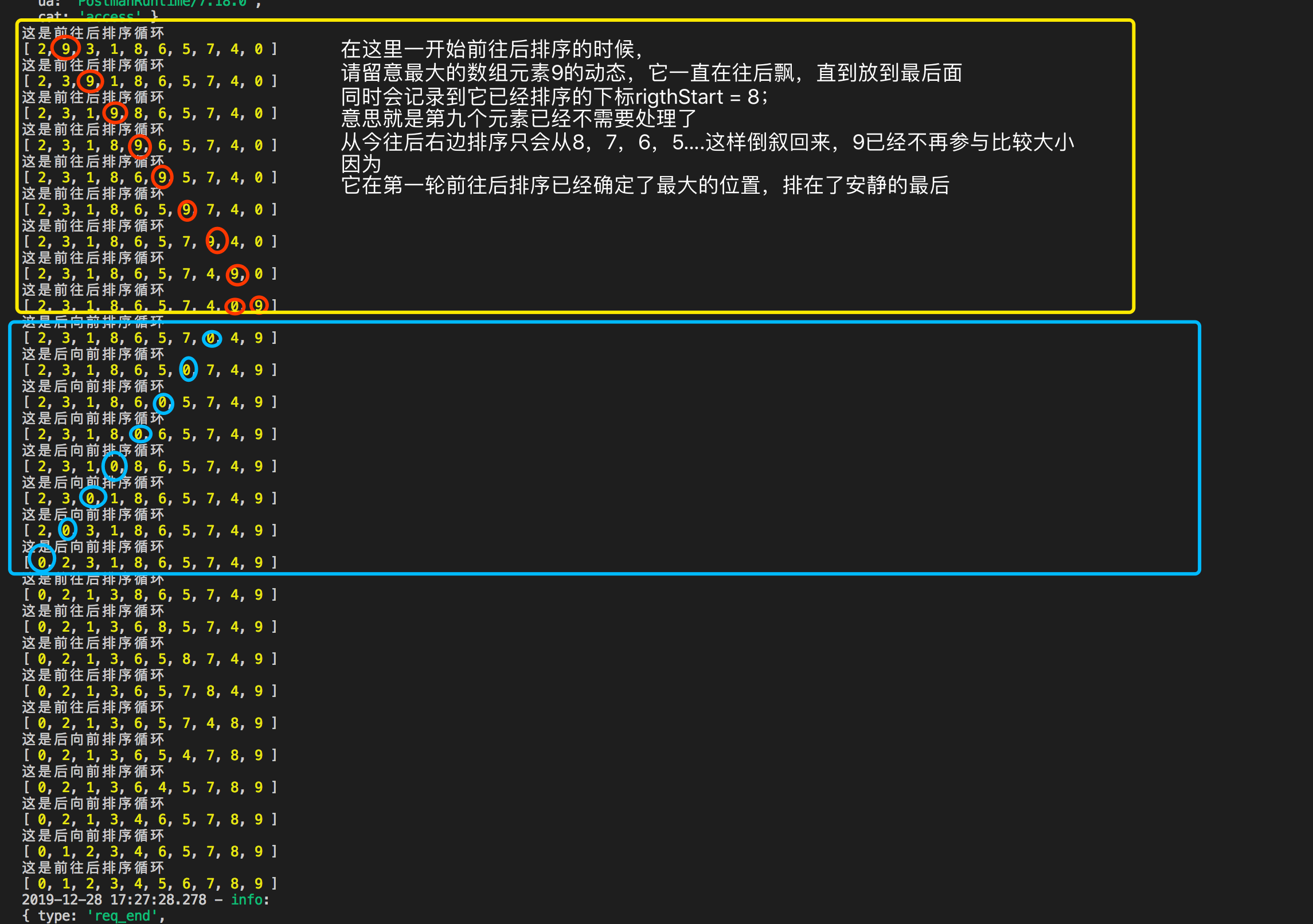可以看到最终结果就是0 1 2 3 4 5 6 7 8 9；排序成功！
双向冒泡排序的坑点：
坑点一：从前往后第一轮容易，从后往前难；记住要准确记录上一次存放好数据的下标，记住那是下一次开始的地方。
坑点二： 只有在不需要再比较和换位置的时候！才能对下标记录赋值！不能把leftStart 和 rightStart 记录下标放在for循环里！不然条件b >= leftStart;之类的地方会永真！一直跑个不停！不要自己挖坑给自己跳！！
坑点三：记住第一轮结束后，右边倒序开始的边界界限就变成了原来的长度-1；第二轮结束后，左边正序的开始边界界限就变成了下标1；不再从下标0开始。这里定义的beginPoint；永远是临时存放左右边界开始的下标！！
// 等待排序的数组
const waitSort = [9, 2, 3, 1, 8, 6, 5, 7, 4, 0];
// 定一个临时容器盒子
let tempBox;

// 左边开始的标志
let leftStart = 0;

// 右边开始的标志
let rightStart = waitSort.length;

// 交汇点
let beginPoint = 0;

// 如果左边跟右边还没接触，证明中间还有未排序的数
while (leftStart < rightStart) {
// 从左边第一个数开始一直比较到最后一个数，找到整个数组中最大那个放到最后
for (let a = leftStart; a < waitSort.length - 1; a++) {
if (waitSort[a] > waitSort[a + 1]) {
tempBox = waitSort[a];
waitSort[a] = waitSort[a + 1];
waitSort[a + 1] = tempBox;
// 循环结束后这里是从后往前的起点，因为a+1已经排好序了是最大的一个数，不需要再挪动
beginPoint = a;
console.log('这是前往后排序循环');
console.log(waitSort);
}
}
// 如果不需要换位置了，则设置右边的起点
rightStart = beginPoint;

// 从后往前找，直到找到整个数组最小的那个放在第一位。
for (let b = rightStart; b >= leftStart; b--) {
if (waitSort[b] < waitSort[b - 1]) {
tempBox = waitSort[b];
waitSort[b] = waitSort[b - 1];
waitSort[b - 1] = tempBox;
// 动
beginPoint = b + 1;
console.log('这是后向前排序循环');
console.log(waitSort);
}
}
// 如果不需要换位置了，则设置左边的起点
leftStart = beginPoint;
}

可能我说的不太清楚，有疑惑的地方可以留言给我，还是记住一定要拿纸拿笔画流程；再自己动手敲一边才会更好理解哈；谢谢大家观察；鞠躬！展开全文java node.js
• 双向冒泡排序算法-Java实现 算法思想： 类似于冒泡排序 首先是定义两个边界即left和right 其次通过left右移进行冒泡排序，即将最左边数据与之后数据的不断比较交换，直到第一个数据为最小，之后left++； right的操作...
双向冒泡排序算法-Java实现
算法思想：
类似于冒泡排序
首先是定义两个边界即left和right
其次通过left右移进行冒泡排序，即将最左边数据与之后数据的不断比较交换，直到第一个数据为最小，之后left++；
right的操作与left类似且对称
结合条件：left>=right时循环中断
代码实现：
public int[] doubleBubbleSort(int []array){
int []arr=array;
int left=0;right=arr.size()-1;
while(left<right){
for(int i=left+1;i<=right;i++){
if(arr[left]>arr[i]){
int tmp=arr[i];
arr[i]=arr[left];
arr[left]=tmp;
}
}
left++;
for(int i=right-1;i>=left;i--){
if(arr[right]<arr[i]){
int tmp=arr[i];
arr[i]=arr[right];
arr[left]=tmp;
}
}
right++;
}
return arr;
}

同样的，该方法对于列表一样适用
只需要调用列表的set方法实现内部数据的调换即可
范例
public List<Student> doubleBubbleSort(List<Student> studentList){
List<Student> list=studentList;
Student student=null;
int left=0,right=studentList.size()-1;
while(left<right)
{
for(int i=left+1;i<=right;i++){
if(list.get(left).getSum()<list.get(i).getSum()){
student=list.get(i);
list.set(i,list.get(left));
list.set(left,student);
}
}
left++;
for(int i=right-1;i>=left;i--){
if(list.get(right).getSum()>list.get(i).getSum()){
student=list.get(i);
list.set(i,list.get(right));
list.set(right,student);
}
}
right--;
}
return list;
}

当然，其中的比较还是数字的比较。


展开全文java
• 冒泡排序：就是按索引逐次比较相邻的两个元素，如果大于/小于(取决于需要升序排还是降序排)，则置换，否则不做改变这样一轮下来，比较了n-1次，n等于元素的个数；n-2, n-3 ... 一直到最后一轮，比较了1次所以比较...
冒泡排序：就是按索引逐次比较相邻的两个元素，如果大于/小于(取决于需要升序排还是降序排)，则置换，否则不做改变这样一轮下来，比较了n-1次，n等于元素的个数；n-2, n-3 ... 一直到最后一轮，比较了1次所以比较次数为递减：从n-1 到 1那么总的比较次数为：1+2+3+...+(n-1),  以等差公式计算：(1+n-1)/2*(n-1) ==> n/2*(n-1) ==> (n^2-n) * 0.5用大O表示算法的时间复杂度：O(n^2) ,  忽略了系数0.5和常数-npublic class BubbleSort {public static void main(String[] args) {int len = 10;int[] ary = new int[len];Random random = new Random();for (int j = 0; j < len; j++) {ary[j] = random.nextInt(1000);}System.out.println("-------排序前------");for (int j = 0; j < ary.length; j++) {System.out.print(ary[j] + " ");}/** 升序， Asc1和Asc2优化了内部循环的比较次数，比较好* 总的比较次数：*   Asc1、Asc2：(1+n-1)/2*(n-1) ==> n/2*(n-1) ==> n*(n-1)/2 ==>(n^2-n)/2*   Asc： n^2-n*///   orderAsc(ary);//   orderAsc2(ary);orderAsc1(ary);//降序，只需要把判断大小于 置换一下}static void orderAsc(int[] ary) {int count = 0;//比较次数int len = ary.length;for (int j = 0; j < len; j++) {//外层固定循环次数for (int k = 0; k < len - 1; k++) {//内层固定循环次数if (ary[k] > ary[k + 1]) {ary[k] = ary[k + 1] + (ary[k + 1] = ary[k]) * 0;//一步交换/* 交换两个变量值* a=a+b* b=a-b* a=a-b*/}count++;}}System.out.println("\n-----orderAsc升序排序后------次数：" + count);for (int j = 0; j < len; j++) {System.out.print(ary[j] + " ");}}static void orderAsc1(int[] ary) {int count = 0;//比较次数int len = ary.length;for (int j = 0; j < len; j++) {//外层固定循环次数for (int k = len - 1; k > j; k--) {//内层从多到少递减比较次数if (ary[k] < ary[k - 1]) {ary[k] = ary[k - 1] + (ary[k - 1] = ary[k]) * 0;//一步交换}count++;}}System.out.println("\n-----orderAsc1升序排序后------次数：" + count);for (int j = 0; j < len; j++) {System.out.print(ary[j] + " ");}}static void orderAsc2(int[] ary) {int count = 0;//比较次数int len = ary.length;for (int j = len - 1; j > 0; j--) {//外层固定循环次数/** k < j; 总的比较次数=(n^2-n)/2*/for (int k = 0; k < j; k++) {//内层从多到少递减比较次数if (ary[k] > ary[k + 1]) {ary[k] = ary[k + 1] + (ary[k + 1] = ary[k]) * 0;//一步交换}count++;}}System.out.println("\n-----orderAsc2升序排序后------次数：" + count);for (int j = 0; j < len; j++) {System.out.print(ary[j] + " ");}}}打印-------排序前------898 7 862 286 879 660 433 724 316 737-----orderAsc1升序排序后------次数：457 286 316 433 660 724 737 862 879 898双向冒泡排序冒泡排序_鸡尾酒排序、就是双向冒泡排序。此算法与冒泡排序的不同处在于排序时是以双向在序列中进行排序,外层比较左右边界l内层一个循环从左向右比，取高值置后；一个循环从右向左，取低值置前；效率上，O(N^2), 不比普通的冒泡快public class Bubble_CocktailSort {public static void main(String[] args) {int len = 10;int[] ary = new int[len];Random random = new Random();for (int j = 0; j < len; j++) {ary[j] = random.nextInt(1000);}/** 交换次数最小也是1次，最大也是(n^2-n)/2次*///   ary=new int[]{10,9,8,7,6,5,4,3,2,1}; //测试交换次数//   ary=new int[]{1,2,3,4,5,6,7,8,10,9}; //测试交换次数System.out.println("-------排序前------");for (int j = 0; j < ary.length; j++) {System.out.print(ary[j] + " ");}orderAsc1(ary);//   orderAsc2(ary);//降序，只需要把判断大小于 置换一下}static void orderAsc1(int[] ary) {int compareCount = 0;//比较次数int changeCount = 0;//交换次数int len = ary.length;int left = 0, right = len -1, tl, tr;while (left < right) {//外层固定循环次数tl = left + 1;tr = right - 1;for (int k = left; k < right; k++) {//内层从多到少递减比较次数, 从左向右if (ary[k] > ary[k + 1]) {//前大于后， 置换ary[k] = ary[k + 1] + (ary[k + 1] = ary[k]) * 0;//一步交换changeCount++;tr = k; //一轮中最后一比较的时候，将k所在索引赋给tr, tr表示以后比较的结束索引值, 从左向右比后，k表示左边的索引}compareCount++;}right = tr;for (int k = right; k > left; k--) {//内层从多到少递减比较次数, 从右向左if (ary[k] < ary[k - 1]) {//后小于前 置换ary[k] = ary[k - 1] + (ary[k - 1] = ary[k]) * 0;//一步交换changeCount++;tl = k; //一轮中最后一比较的时候，将k所在索引赋给tl, tl表示以后比较的开始索引值, 从向右向左比后，k表示右边的索引}compareCount++;}left = tl;}System.out.println("\n-----orderAsc1升序排序后------比较次数：" + compareCount + ", 交换次数：" + changeCount);for (int j = 0; j < len; j++) {System.out.print(ary[j] + " ");}}//跟orderAsc1的思路没有区别static void orderAsc2(int[] ary) {int compareCount = 0;//比较次数int changeCount = 0;//交换次数int len = ary.length;int l = 0, r = len -1, tl, tr;for (; l < r; ) {//外层固定循环次数tl = l + 1;tr = r - 1;/** 从左向右比，将大的移到后面*/for (int k = l; k < r; k++) {if (ary[k] > ary[k + 1]) {int temp = ary[k] + ary[k + 1];ary[k + 1] = temp - ary[k + 1];ary[k] = temp - ary[k + 1];changeCount++;tr = k;}compareCount++;}r = tr;/** 从右向左比，将小的移到前面*/for (int k = r; k > l; k--) {if (ary[k] < ary[k - 1]) {int temp = ary[k] + ary[k - 1];ary[k - 1] = temp - ary[k - 1];ary[k] = temp - ary[k - 1];changeCount++;tl = k;}compareCount++;}l = tl;}System.out.println("\n-----orderAsc2升序排序后------比较次数：" + compareCount + ", 交换次数：" + changeCount);for (int j = 0; j < len; j++) {System.out.print(ary[j] + " ");}}}打印-------排序前------783 173 53 955 697 839 201 899 680 677-----orderAsc1升序排序后------比较次数：34, 交换次数：2253 173 201 677 680 697 783 839 899 955
展开全文• java实现冒泡排序 一、冒泡排序： 利用冒泡排序对数组进行排序 二、基本概念： 依次比较相邻的两个数，将小数放在前面，大数放在后面。即在第一趟：首先比较第1个和第2个数，将小数放前，大数放后。然后比较第2个数...
java实现冒泡排序
一、冒泡排序：
利用冒泡排序对数组进行排序
二、基本概念：
依次比较相邻的两个数，将小数放在前面，大数放在后面。即在第一趟：首先比较第1个和第2个数，将小数放前，大数放后。然后比较第2个数和第3个数，将小数放前，大数放后，如此继续，直至比较最后两个数，将小数放前，大数放后。至此第一趟结束，将最大的数放到了最后。在第二趟：仍从第一对数开始比较（因为可能由于第2个数和第3个数的交换，使得第1个数不再小于第2个数），将小数放前，大数放后，一直比较到倒数第二个数（倒数第一的位置上已经是最大的），第二趟结束，在倒数第二的位置上得到一个新的最大数（其实在整个数列中是第二大的数）。如此下去，重复以上过程，直至最终完成排序。
三、实现思路：
用二重循环实现，外循环变量设为i，内循环变量设为j。假如有n个数需要进行排序，则外循环重复n-1次，内循环依次重复n-1，n-2，…，1次。每次进行比较的两个元素都是与内循环j有关的，它们可以分别用a[j]和a[j+1]标识，i的值依次为1,2,…,n-1，对于每一个i，j的值依次为0,1,2,…n-i 。
设数组长度为N：
1．比较相邻的前后二个数据，如果前面数据大于后面的数据，就将二个数据交换。
2．这样对数组的第0个数据到N-1个数据进行一次遍历后，最大的一个数据就“沉”到数组第N-1个位置。
3．N=N-1，如果N不为0就重复前面二步，否则排序完成。
package 冒泡排序;

import java.util.Arrays;
/**
* 冒泡排序
* @author zy
*
*/
public class BubbleSort {
public static void BubbleSort(int[] arr) {
int temp;//定义一个临时变量
for(int i=0;i<arr.length-1;i++){//冒泡趟数
for(int j=0;j<arr.length-i-1;j++){
if(arr[j+1]<arr[j]){
temp = arr[j];
arr[j] = arr[j+1];
arr[j+1] = temp;
}
}
}
}
public static void main(String[] args) {
int arr[] = new int[]{1,6,2,2,5};
BubbleSort.BubbleSort(arr);
System.out.println(Arrays.toString(arr));
}
}

五、性能分析：
若记录序列的初始状态为"正序"，则冒泡排序过程只需进行一趟排序，在排序过程中只需进行n-1次比较，且不移动记录；反之，若记录序列的初始状态为"逆序"，则需进行n(n-1）/2次比较和记录移动。因此冒泡排序总的时间复杂度为O(n*n)。
六、算法优化：
冒泡排序法存在的不足及改进方法：
第一，在排序过程中，执行完最后的排序后，虽然数据已全部排序完备，但程序无法判断是否完成排序，为了解决这一不足，可设置一个标志位flag，将其初始值设置为非0，表示被排序的表是一个无序的表，每一次排序开始前设置flag值为0，在进行数据交换时，修改flag为非0。在新一轮排序开始时，检查此标志，若此标志为0，表示上一次没有做过交换数据，则结束排序；否则进行排序；
package 冒泡排序;
import java.util.Arrays;
/**
* 冒泡排序改进版
* @author zy
*
*/
public class BubbleSort1 {
public static void BubbleSort(int[] arr) {
boolean flag = true;
while(flag){
int temp;//定义一个临时变量
for(int i=0;i<arr.length-1;i++){//冒泡趟数，n-1趟
for(int j=0;j<arr.length-i-1;j++){
if(arr[j+1]<arr[j]){
temp = arr[j];
arr[j] = arr[j+1];
arr[j+1] = temp;
flag = true;
}
}
if(!flag){
break;//若果没有发生交换，则退出循环
}
}
}
}
public static void main(String[] args) {
int arr[] = new int[]{1,6,2,2,5};
BubbleSort.BubbleSort(arr);
System.out.println(Arrays.toString(arr));
}
}

第二、在冒泡排序中，一趟扫描有可能无数据交换，也有可能有一次或多次数据交换，在传统的冒泡排序算法及近年来的一些改进的算法中，只记录一趟扫描有无数据交换的信息，对数据交换发生的位置信息则不予处理。为了充分利用这一信息，可以在一趟全局扫描中，对每一反序数据对进行局部冒泡排序处理，称之为局部冒泡排序。局部冒泡排序与冒泡排序算法具有相同的时间复杂度，并且在正序和逆序的情况下，所需的关键字的比较次数和移动次数完全相同。由于局部冒泡排序和冒泡排序的数据移动次数总是相同的，而局部冒泡排序所需关键字的比较次数常少于冒泡排序，这意味着局部冒泡排序很可能在平均比较次数上对冒泡排序有所改进，当比较次数较少的优点不足以抵消其程序复杂度所带来的额外开销，而当数据量较大时，局部冒泡排序的时间性能则明显优于冒泡排序。对于N个无序数据，我们在进行一趟冒泡排序时，如果第k个数据和第k+1个数据逆序，那么对第k+1个数据进行一趟向前的冒泡排序，使其移动到合适的位置，也就是说让前面k+1个数据调节为正序。因为这种冒泡法只对前k+1个数据冒泡处理，所以我们称它为——局部冒泡
Java 实现双向冒泡排序
冒泡排序_鸡尾酒排序
就是双向冒泡排序
此算法与冒泡排序的不同处在于排序时是以双向在序列中进行排序,外层比较左右边界l<r,
内层一个循环从左向右比，取高值置后；一个循环从右向左，取低值置前；
效率上，O(N^2), 不比普通的冒泡快
public class Bubble_CocktailSort {
public static void main(String[] args) {
int len = 10;
int[] ary = new int[len];
Random random = new Random();
for (int j = 0; j < len; j++) {
ary[j] = random.nextInt(1000);
}
/*
* 交换次数最小也是1次，最大也是(n^2-n)/2次
*/
//		ary=new int[]{10,9,8,7,6,5,4,3,2,1}; //测试交换次数
//		ary=new int[]{1,2,3,4,5,6,7,8,10,9}; //测试交换次数
System.out.println("-------排序前------");
for (int j = 0; j < ary.length; j++) {
System.out.print(ary[j] + " ");
}

orderAsc1(ary);
//		orderAsc2(ary);

//降序，只需要把判断大小于 置换一下

}

static void orderAsc1(int[] ary) {
int compareCount = 0;//比较次数
int changeCount = 0;//交换次数
int len = ary.length;
int left = 0, right = len -1, tl, tr;
while (left < right) {//外层固定循环次数
tl = left + 1;
tr = right - 1;
for (int k = left; k < right; k++) {//内层从多到少递减比较次数, 从左向右
if (ary[k] > ary[k + 1]) {//前大于后， 置换
ary[k] = ary[k + 1] + (ary[k + 1] = ary[k]) * 0;//一步交换
changeCount++;
tr = k; //一轮中最后一比较的时候，将k所在索引赋给tr,  tr表示以后比较的结束索引值,  从左向右比后，k表示左边的索引
}
compareCount++;
}
right = tr;
for (int k = right; k > left; k--) {//内层从多到少递减比较次数, 从右向左
if (ary[k] < ary[k - 1]) {//后小于前 置换
ary[k] = ary[k - 1] + (ary[k - 1] = ary[k]) * 0;//一步交换
changeCount++;
tl = k; //一轮中最后一比较的时候，将k所在索引赋给tl,  tl表示以后比较的开始索引值, 从向右向左比后，k表示右边的索引
}
compareCount++;
}
left = tl;
}
System.out.println("\n-----orderAsc1升序排序后------比较次数：" + compareCount + ", 交换次数：" + changeCount);
for (int j = 0; j < len; j++) {
System.out.print(ary[j] + " ");
}
}

//跟orderAsc1的思路没有区别
static void orderAsc2(int[] ary) {
int compareCount = 0;//比较次数
int changeCount = 0;//交换次数
int len = ary.length;
int l = 0, r = len -1, tl, tr;
for (; l < r; ) {//外层固定循环次数
tl = l + 1;
tr = r - 1;
/*
* 从左向右比，将大的移到后面
*/
for (int k = l; k < r; k++) {
if (ary[k] > ary[k + 1]) {
int temp = ary[k] + ary[k + 1];
ary[k + 1] = temp - ary[k + 1];
ary[k] = temp - ary[k + 1];
changeCount++;
tr = k;
}
compareCount++;
}
r = tr;
/*
* 从右向左比，将小的移到前面
*/
for (int k = r; k > l; k--) {
if (ary[k] < ary[k - 1]) {
int temp = ary[k] + ary[k - 1];
ary[k - 1] = temp - ary[k - 1];
ary[k] = temp - ary[k - 1];
changeCount++;
tl = k;
}
compareCount++;
}
l = tl;
}
System.out.println("\n-----orderAsc2升序排序后------比较次数：" + compareCount + ", 交换次数：" + changeCount);
for (int j = 0; j < len; j++) {
System.out.print(ary[j] + " ");
}
}
}



展开全文数据结构 算法 java
• 以整数升序排序为例来简单说明一下双向冒泡排序的过程：首先从前往后把最大数移到最后，然后反过来从后往前把最小的一个数移动到数组最前面，这一过程就是第一轮，然后重复这一过程，最终就会把整个数组从小到大排列...
• 冒泡排序算法步骤：比较相邻的元素，如果第一个比第二个大，就交换他们两个的位置；对每对相邻的元素执行同样的操作，这样一趟下来，最后的元素就是最大的；除了已得出来的最大元素，把剩余的元素重复前面步骤，直到...PHP
• 用10万个int数据对两个冒泡算法进行测试，测试结果：单向冒泡平均需要60s的时间，而双向冒泡平均需要30s的时间。测试发现，双向冒泡确实可以提高排序效率。
• //计数排序 TypeName void Sort(T R[],int n) { int l = 0, r = n - 1; while (l) { for (int i = l; i ; i++)//由左向右扫描，将最大值置于右边 { if (R[i]>R[i + 1]) SWAP(R, i, i + 1); } --r;...
• 以整数升序排序为例来简单说明一下双向冒泡排序的过程：首先从前往后把最大数移到最后，然后反过来从后往前把最小的一个数移动到数组最前面，这一过程就是第一轮，然后重复这一过程，最终就会把整个数组从小到大排列...
•  冒泡排序算法的运作如下：（从后往前）  1.比较相邻的元素。如果第一个比第二个大，就交换他们两个。  2.对每一对相邻元素作同样的工作，从开始第一对到结尾的最后一对。在这一点，最后的元素应该会是最大的数...C++
• [b]以下是一个双向冒泡排序算法[/b] int high = table.length; int left = 1; int right = high - 1; int t = 0; do { //正向部分 for (int i = right; i >= left; i--) { if ...java
• 本文源自微信公众号【Python编程和深度学习】原文链接：经典排序算法和python详解(二)：冒泡排序、双向冒泡排序、插入排序和希尔排序，欢迎扫码关注鸭！目录一、冒泡排序(Bubble Sort)二、冒泡排序法改进三、双向...
• **排序算法**应该算是一个比较热门的话题，在各个技术博客平台上也都有一些博文进行了一定程度的讲解。...本文就先从最简单的冒泡排序开始说起，别说你已经彻底了解了冒泡排序算法（虽然一开始我也是这样以为的）。算法
• 本文源自微信公众号【Python编程和深度学习】原文链接：经典排序算法和python详解(二)：冒泡排序、双向冒泡排序、插入排序和希尔排序，欢迎扫码关注鸭！目录一、冒泡排序(Bubble Sort)二、冒泡排序法改进三、双向...python排序...